# The Second Order: Linear Models in a Nonlinear Valuation Process

###### Fixed Income

By Bill McCoy  |  July 28, 2015

Equities have long employed the use of linear valuation models, starting with the Capital Asset Pricing Model: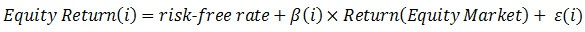In this framework, β(i) is the systematic factor exposure, and ε(i) is the idiosyncratic return for any security i. This framework has grown to where there are many factors driving return. The theory assumes that the relationship between the underlying factors and equity return is linear in nature. It is important to note that the linearity is assumed rather than derived, and thus assumes that nonlinear effects are minor.

Equity linear models migrated from valuation into equity risk measurement. What is interesting is how linear models continued to migrate over to fixed income risk measurement, typically of the form: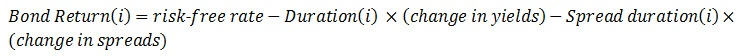However, fixed income valuation is nonlinear by definition, and the nonlinear effects are not minor. The dependence of price on yield and spread is related by the nonlinear equation: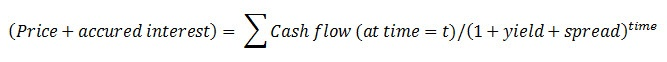Mathematically, the Taylor series expansion relates mathematical derivatives to a change in yield or spread. Duration is the first derivative of percentage change in price given a change in yield or spread, and the second derivative is convexity. Bond return can then be written as the non-linear equation: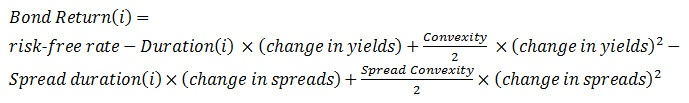To demonstrate the impact of the nonlinear convexity terms, consider the graph below: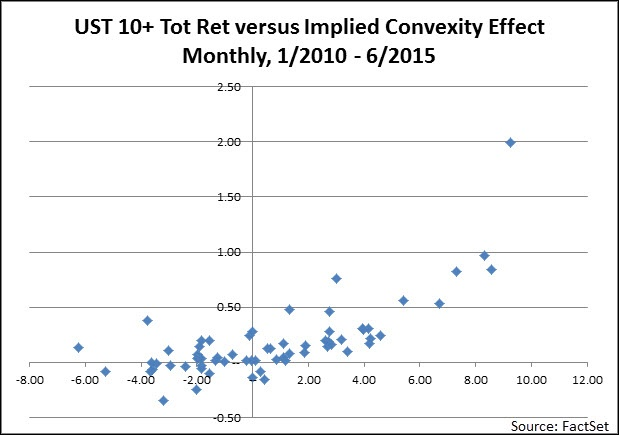This is a plot of the monthly total return of a U.S. Treasury 10-year+ index versus the estimated nonlinear components of return. By nonlinear components we mean the monthly return less the monthly yield and the duration times the change in yield. In terms of a formula, it is: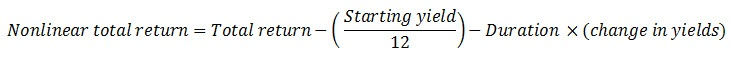What is interesting to note is the upward facing bowl shape. Convexity slows the price change implied by duration during periods when interest rates rise and accelerates the impact implied by duration during periods when interest rates decline. For extreme negative or positive returns, the impact of convexity is even more pronounced and suggests linear risk measures are overestimating risk.

As expected, a similar effect is present in spread return. Below is a plot of the monthly spread return of a U.S. Corporate BBB index versus the estimated nonlinear components of spread return. By nonlinear components we mean the monthly spread return less the spread duration times the change in spread. In terms of a formula, it is: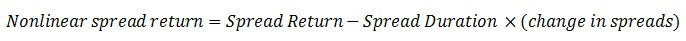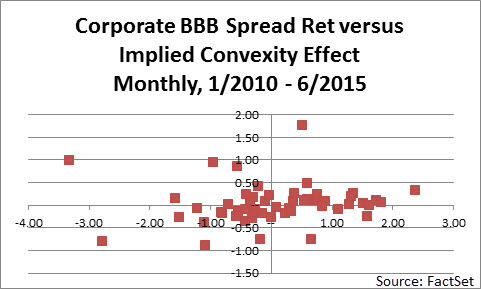We should note that for negatively convex sectors, such as mortgages, the bowl is downward facing and suggests that linear risk measures are underestimating risk.

The impact on risk management can be estimated. Let's return to the U.S. Treasury 10-year+ index and measure the daily VaR versus the actual daily returns at different confidence intervals over the past two years. The number of breeches is a measure of the effectiveness of the estimated VaR. By ignoring the nonlinear return effects, the number of breeches increases, thus confirming the overestimation of risk, as shown below: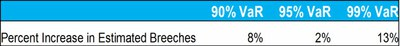In summary, convexity and other nonlinear effects are not minor in fixed income portfolio management, and in fact are actively managed. Nonlinear effects should play an equally large role in fixed income risk management.

Read more about fixed income and multi-asset class portfolio management in our eBook: Understanding Risks in a Global Multi-Asset Class Portfolio.

## Bill McCoy

###### Vice President, Senior Director, Analytics Strategy

Bill has written and spoken extensively on fixed income hedging and return attribution. He has earned a Master degree in Operations Research from the University of North Carolina and is a Chartered Financial Analyst and Professional Risk Manager.